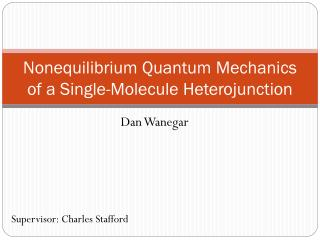DownloadDownload PresentationNonequilibrium Quantum Mechanics of a Single-Molecule Heterojunction

# Nonequilibrium Quantum Mechanics of a Single-Molecule Heterojunction

Download Presentation## Nonequilibrium Quantum Mechanics of a Single-Molecule Heterojunction

- - - - - - - - - - - - - - - - - - - - - - - - - - - E N D - - - - - - - - - - - - - - - - - - - - - - - - - - -
##### Presentation Transcript

1. Nonequilibrium Quantum Mechanics of a Single-Molecule Heterojunction Dan Wanegar Supervisor: Charles Stafford

2. The Problem Finding the electron transport through a single-molecule junction. Electrons, as fermions, obey the Pauli Exclusion Principle, so learning creation and annihilation operators was necessary. The Green’s function, G, which describes the quantum dynamics, was the next step. Physics observables, such as electric current, can be expressed in terms of G

3. The Hamiltonian H=Hmol+Hleads+HT d and d+ are creation and annihilation operators obeying: {di,dj+}=didj++dj+di=di,j {di,dj}={di+,dj+}=0 (Hmol)ij = hijdi+dj (Hleads)k = ekck+ck (HT)nk=Vn,kdn+ck+Vn,k*ck+dn

4. The Retarded Green’s Function Plugging in previous equations followed by a Fourier transform (right) gives G = [IE – Hmol – S]-1, where Si,j = The i0+ ensures a retarded function as opposed to advanced In the broad-band limit, Ga = i(Sr – Sa) = And Sr = Sa* is taken to be imaginary, so Sr = -iGa/2

5. Current and The Transmission Matrix • The probability of transmission from lead i to lead j is given by the i,j component of the transmission matrix. • The current into lead i is given by • The transmission matrix is given by Tij = Tr(GiGrGjGa) • A proof can be found in Electronic Transport in Mesoscopic Systems by Supriyo Datta

6. The General Diatomic Conductor When G1, G2, and G are entirely arbitrary, the matrix multiplication gives: T12=SijkmG1ijG2kmGjkGim* Which can be expanded and simplified due to symmetries to: T12 = Sij |G1ii||G2jj||Grij| +2Re(G111G212Gr11*Gr12 + G112G211Gr11*Gr12 + G112G212Gr12*Gr21 + G112G221Gr11*Gr22 + G112G222Gr12*Gr22 + G122G212Gr22*Gr21) In general, the G112 and G212 terms are small enough and complex, so the nasty part usually averages to zero.

7. The Vertical Diatomic Conductor:FanoAntiresonances An interesting case to note is the G11.1 and G21.1 being the nonzero in the connection terms. This gives G-1= Since G111 and G211 are the only nonzero terms, this means that T11 = G1G2|G11|2, Which has exactly one node at E=e2. This can also be looked at as an atomic conductor (atom 1) with three leads (atom 2 being the 2nd). This makes G =

8. The Horizontal Diatomic Conductor:Exceptional Points For a horizontal conductor, the time-dependant transmission function is  where So if e1 = e2, Which is either purely imaginary or purely real. As can be seen above, there is a very large difference between 2V>|G’| and 2V<|G’|, so |G’|=2V is called the exceptional point. So how does this relate to the energy-dependant transmission function?  D.M.Cardamone, C.A.Stafford, and B.R.Barrett, Phys. Stat. Sol. (b) 230, No. 2 (2002)

9. What About The Energy-Dependant Transmission Function ? Using the energy-dependant Green’s function from earlier, T12= G111G211|G12|2, and Which is especially interesting to look at when e1=e2, as it turns into DE =

10. A Less Distinguishable Result DE / G = 2.3 DE / G = 1.2 For large enough real DE and small enough G, the transmission function has two peaks. Otherwise, only one peak is observed. However, E ± lies in the complex plane, but E can only be real. This means that peaks don’t occur at exactly E = Re(E ±). So for small enough DE and large enough G, the transmission function doesn’t appear to be qualitatively different for DE real or imaginary. DE / G = .59 DE / G = .22

11. Taking the n-th Derivative… DE / G = 2.3 1.2 .59 .22 Shows that even for very small real DE, there is still a qualitative difference from imaginary DE. n=0 1 2 5

12. Conclusions The nonequilibrium quantum dynamics of electrons in a single-moleule junction can be solved exactly using Green’s functions when neglecting electron-electron interactions For a diatomic molecule, both a Fano antiresonance and an exceptional point can occur, depending on the geometry.